# Test: Quantitative Techniques- 8

## 20 Questions MCQ Test IBPS PO Prelims- Study Material, Online Tests, Previous Year | Test: Quantitative Techniques- 8

Description
Attempt Test: Quantitative Techniques- 8 | 20 questions in 30 minutes | Mock test for Banking Exams preparation | Free important questions MCQ to study IBPS PO Prelims- Study Material, Online Tests, Previous Year for Banking Exams Exam | Download free PDF with solutions
QUESTION: 1

### A fan is listed at Rs1500 and A discount of 20% is offered at the listed price. What additional discount must be offered to bring net price to Rs 1104?

Solution:

M.P → Rs 1500
After 1st discount → 80% of 1500 = 1200
For S.P to be 1104, additional RS 96 discount required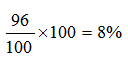QUESTION: 2

Solution:
QUESTION: 3

### A thief is spotted by a policeman, from a distance of 200m.the policeman and thief start running at the speed of 20kmph and 16kmph. How far the police man will have to run so as to catch the thief

Solution:

Net advantage = 4 Km is 1 hr.
i.e. 4000 m in 1 hr.
So, for 200 m advantage time required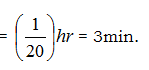Now, Policeman runs 20 km in 1 hr.
So in 3 min he will run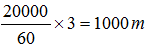QUESTION: 4

If the radius of a circle is increased by 50% then the area is increased by?

Solution: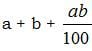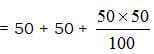= 100 + 25 = 125%

QUESTION: 5

If 0.06% of a number is 84, then 30% of that number is?

Solution:

Let the no. be x
x*6/100 =84
x=84*100/6
=14*100
=1400
30% of 1400 is
1400*30/100
14*30
=420

QUESTION: 6

A sum was divided among P, Q, R. R got double than P, who got double than Q. If the difference between the shares of Q and R is Rs 3675, then the sum was?

Solution:

R : P  2 : 1   R : P : Q  Diff (R,Q)
P : Q  2 : 1 →   3675
3 ≡ 3675
1 ≡ 1225
Sum > ≡ 1225 x 7 = 8575

QUESTION: 7

One side of rectangular field is 15m and one of the diagonal is 17m. Find the area of the field

Solution: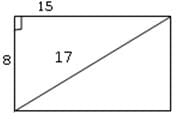Area = 15 x 8 = 120 m2

QUESTION: 8

The denominator of a fraction is greater than it’s numerator by 11. If 8 is added to both it’s numerator and denominator, then it becomes 3/4 the fraction is?

Solution:
QUESTION: 9

The difference of two number is 11 and one-fifth of their sum is 9 find the numbers?

Solution:

28 + 17 = 45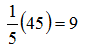QUESTION: 10

The average of five consecutive even numbers A, B, C, D and E is 96. What is the product of A and E?

Solution: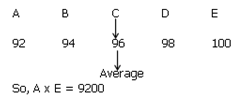QUESTION: 11

The difference between the age of two person is 8 years. 12 Years ago, the elder one was twice the age of younger one. What is the ratio of their present ages?

Solution: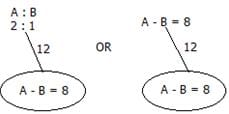So, 1 ≡ 8yrs
2 ≡ 16 yrs
So ratio of their present ages = 20 : 28 = 5 : 7

QUESTION: 12

40% of the greater number is equal to 60% of the smaller. If their sum is 150 then the greater no. is?

Solution:

40% of G = 60% S,   Now G : S = 3 : 2
3 + 2 ≡ 150   so 5 ≡ 150
1 ≡ 30
So 3 ≡ 90

QUESTION: 13

Successive discount of 10% and 20% is equivalent to single discount of ?

Solution: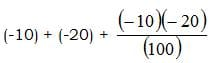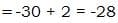QUESTION: 14

Two metals contain zinc and copper in the ratio 2:1 and 4:1 respectively. In what ratio these two must be mixed to get a new metal containing zinc and copper in the ratio 3:1?

Solution: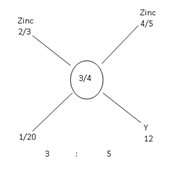QUESTION: 15

Alok started a business with a sum of rs.50000. After a year, he again invested Rs 30000 and made Sudhir his partner who invested Rs 70000. At the end of the 3 years, total profit was Rs 87500. What is the share of Sudhir?

Solution:

A : S
(50,000 x 1) (70,000 x 2) (80,000 x 2)
2,10,000 1,40,000 Sum
3 : 2 → 87500
5 ≡ 87500
1 ≡ 17500
2 ≡ 35000

QUESTION: 16

Two trains 128 meters and 122 meters long are running towards each other on parallel lines at 48 kmph and 42kmph respectively. In what time will they clear off each other from the moment they meet?

Solution:
D = 120 + 80 = 200 M
S = 72 km/h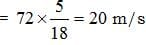T = 20/20 = 1 sec
QUESTION: 17

Pipe A can fill the tank in 3hrs and pipe B can fill the tank in 4hrs. An outlet pipe C can empty the tank in 6 hrs. If all pipes are kept open simultaneously, in how many hours will the tank be half full?

Solution:

Tank capacity = 12 L
A + B → 7L in 1 min
C → -2L in 1 min
So Net result = 5L in 1min
So, time taken = 12/5

QUESTION: 18

In how many years a certain sum doubles itself at 4% per annum simple interest ?

Solution:

P = 100, S.I = 100, R = 4%, t = ??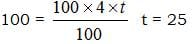QUESTION: 19

I can row 40km downstream and 28km upstream taking 10hours each time-what is the speed of current?

Solution:

Speed downstream = 40/10 = 4hrs
Speed upstream = 28/10 = 2.8 hrs
Spees upstream  = 4-2.8/2
= 1.2/2 = 0.6

QUESTION: 20

Three numbers are in the ratio 1:2:3 and their HCF is 12.the numbers are?

Solution:

The correct option is D.
The HCF in x, 2x and 3x is x because 1, 2, 3 are prime. Hence, x = 12; then the other numbers are 24 and 36.Use Code STAYHOME200 and get INR 200 additional OFF Use Coupon Code